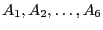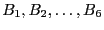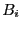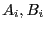Next: A robot control problem Up: Examples of applications of Previous: A geometrical example   Contents

A robot kinematics example

We present here a simplified version of the problem (see  for a more detailed version).

Consider a plane in the 3D space, called the base, on which lie 6 pointswhose coordinates in an arbitrary reference frame.

Consider another plane, called the platform, on which lie similarly 6 points. To this plane we attach a frame, called the mobile frame. The coordinates of the 6 pointsin the mobile frame are supposed to be known. These 6 points constitute a rigid body which has a position/orientation with respect to the reference frame (i.e the location of any point of the rigid body may be calculated as soon as we know the coordinates of a point of this body in the reference frame and the rotation matrix that relates the mobile frame to the reference frame).

Assume now that the distances between each pairis fixed and is known. The problem is to determine what are the possible position/orientation of the rigid body such that these distance constraints are satisfied.

This problem is very important in practice. The platform is the "hand" of the robot and the distances between themay be changed by using actuators. The only information we have in the robot is obtained through sensors that measure the distances between the. Solving the above problem allows one to determine where is the hand of the robot by using the sensory information (but this problem has also other application such as robot calibration).

This problem is called the direct kinematics problem. It has a counterpart, the inverse kinematics problem: being given the position/orientation of the rigid body computes the distances between the pairs. This inverse kinematics problem has a straightforward solution: each squared distance can be calculated as a function of the parameters that describe the position/orientation of the rigid body. Hence solving the direct kinematic problem is equivalent to solve the system of equations of the inverse kinematics in the position/orientation parameters.

This problem is considered as one of the most difficult robot kinematics problem. Before 1992 the only known method to solve this problem was to use the Newton scheme and there was no theoretical result on this problem. Afterward with the use of sophisticated mathematics it has been possible to show theoretical results:

• there will be at most 40 solutions, complex and real
• examples with 40 real solutions have been exhibited
• it is possible to reduce this problem to the the solving of a 40th order univariate polynomial
But still was lacking a practical algorithm to compute the solutions: indeed the manipulation needed to get the 40th order polynomial are difficult to automatize.

The fastest algorithm to solve this problem is the use of the Gröebner basis package of Faugère, Rouillier that allow to compute all the solutions in a computation time that ranges between a few seconds to less than 30 seconds.

Interval analysis allows to solve this problem. Basically we have noticed that we may choose as unknowns the coordinates of 3 points on the platform (called the reference points). Indeed the coordinates of the 3 other points may be obtained as linear combination of the 3 chosen points (i.e. they are virtual points). Furthermore the only equations we have are only distance equations: distances between the pairsand distances between the reference points and the virtual points. Hence the distance solving algorithm described in section 2.16 is convenient for this problem.

Trials have shown that the computation time ranges between 10 to 40 seconds, which is competitive with the algorithm of Faugère, Rouillier. Furthermore in most practical cases we are not interested in computing all the solutions but only the one that corresponds to the actual position/orientation of the platform and as the direct kinematics problem has to be solved almost continuously and the robot speed is limited we may determine a small search domain in which the current solution should be located. In that case the interval analysis based algorithm is very fast as it is sensitive to the search space (at the opposite of the Gröebner approach that has to compute all the solutions and then sort which one is the current solution and hence is working in a constant time). The algorithm is also safe compared to the Newton scheme that may converge toward a solution that is not the current one. Indeed we may guarantee that the current solution is included in the algorithm result. Furthermore if more than one solution is found then it is better to stop immediately the robot as the result of the calculation is used to control it.Next: A robot control problem Up: Examples of applications of Previous: A geometrical example   Contents
Jean-Pierre Merlet 2012-12-20